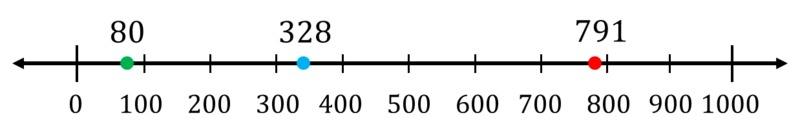# Rounding to the Nearest 100 and 1000

Alignments to Content Standards: 3.NBT.A.1 4.NBT.A.3

Plot the following numbers on the number line:

80

328

7911. Round each number to the nearest 100. How can you see this on the number line?

2. Round each number to the nearest 1000. How can you see this on the number line?

## IM Commentary

Part (a) of this task fits squarely within third grade when students "use place value understanding to round whole numbers to the nearest 10 or 100" (3.NBT.A.1). Part (b) is a first step in rounding beyond tens and hundreds. This task naturally builds off of 3.NBT Rounding to the Nearest Ten and Hundred in third grade. Since fourth graders are expected to "use place value understanding to round multi-digit whole numbers to any place" (4.NBT.A.3), this task also naturally builds towards 4.NBT Rounding to the Nearest 1000. As such, it is a good task for both third and fourth graders.

## Solution

The three numbers are plotted on the number line below:For rounding to the nearest 100 we find which two hundreds the number lies between and round to the nearer of the two. Similarly, for rounding to the nearest 1000, we find the two thousands the number lies between (0 and 1000 for all of these numbers) and round to the nearer of the two.

1. 80 lies between 0 and 100. 50 is exactly half-way between 0 and 100, and 80 is between 50 and 100. So 80 is closer to 100 than 0 on the number line and it rounds up to 100.

328 lies between 300 and 400. 350 is exactly half-way between 300 and 400, and 328 is between 300 and 350. So 328 is closer to 300 than 400 on the number line and it rounds down to 300.

791 lies between 700 and 800. 750 is exactly half-way between 700 and 800, and 791 is between 750 and 800. So 791 is closer to 800 than 700 on the number line and it rounds up to 800.

2. 500 is exactly half-way between 0 and 1000, and 80 is between 0 and 500. So 80 is closer to 0 than 1000 on the number line and it rounds down to 0.

500 is exactly half-way between 0 and 1000, and 328 is between 0 and 500. So 328 is closer to 0 than 1000 on the number line and it rounds down to 0.

500 is exactly half-way between 0 and 1000, and 791 is between 500 and 1000. So 791 is closer to 1000 than 0 on the number line and it rounds up to 1000.